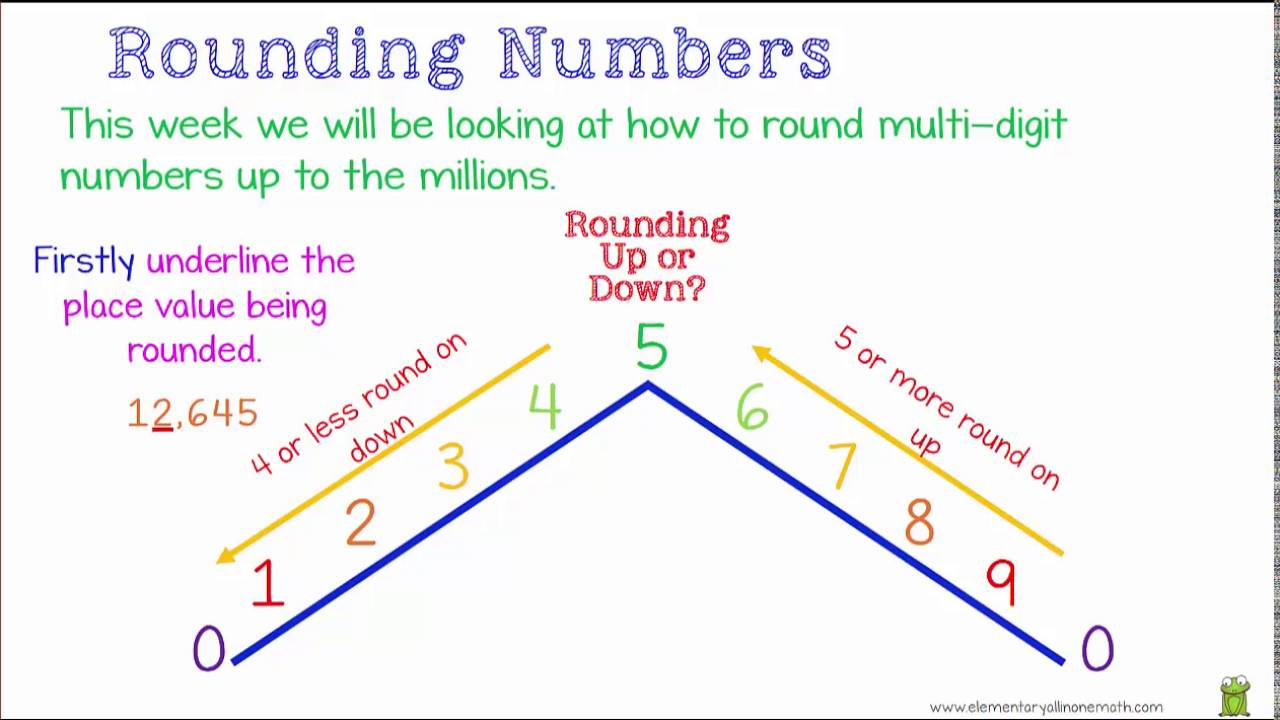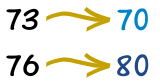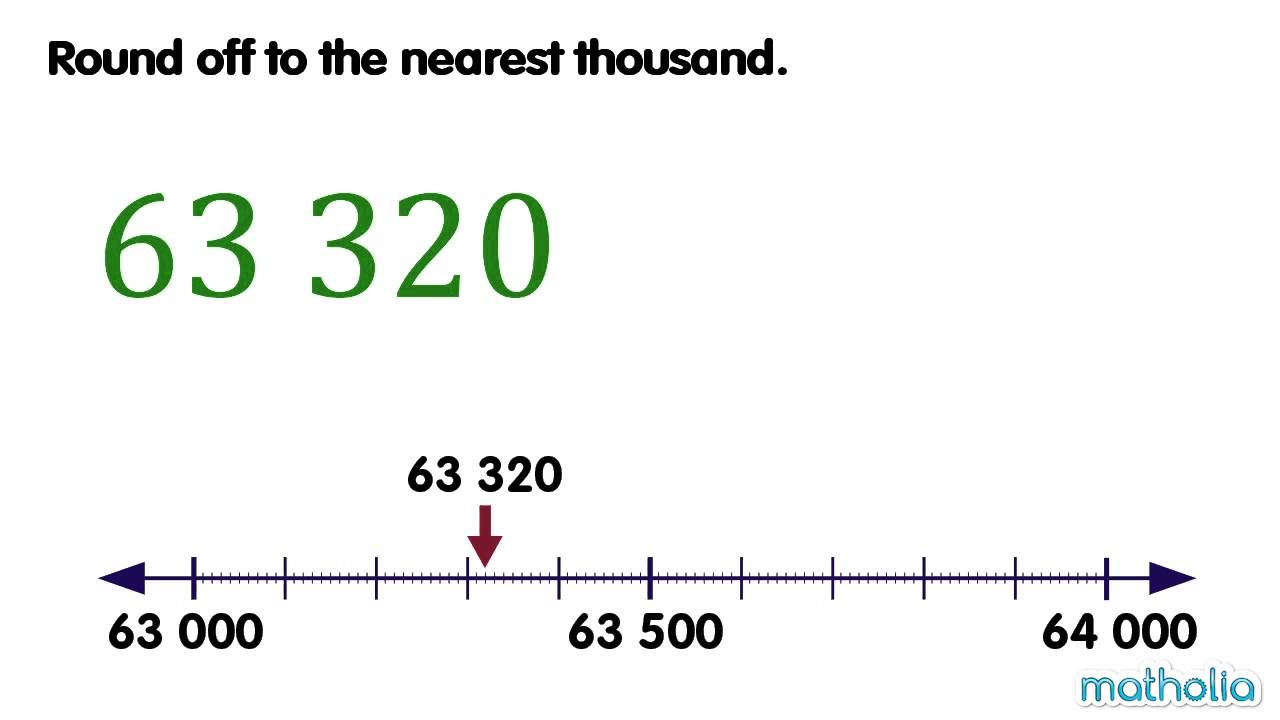Sunday, 6 October 2019

ROUNDING NUMBERS

What is "Rounding" ?How to roud numbers to the nearest ten:

Make the numbers that end in 1 through 4 into the next lower number that ends in 0. For example 74 rounded to the nearest ten would be 70.
Numbers that end in a digit of 5 or more should be rounded up to the next even ten. The number 88 rounded to the nearest ten would be 90.Example: 73 rounded to the nearest ten is 70, because 73 is closer to 70 than to 80. But 76 goes up to 80.

How to roud numbers to the nearest hundred:

To round numbers to the nearest hundred, make the numbers that end in 1 through 49 into the next lower number that ends in 00. For example 424 rounded to the nearest hundred would be 400. Numbers that have the last two digits of 50 or more should be rounded up to the next even hundred. The number 988 rounded to the nearest hundred would be 1000.How to roud numbers to the nearest thousand:

To round numbers to the nearest thousand, make the numbers whose last three digits are 001 through 499 into the next lower number that ends in 000. For example, 6424 rounded to the nearest thousand is 6000. Numbers that have the last three digits of 500 or more should be rounded up to the next even thousand. The number 8788 rounded to the nearest thousand is 9000.Friday, 27 September 2019

MY NEIGHBOUR'S DOG IS PURPLE